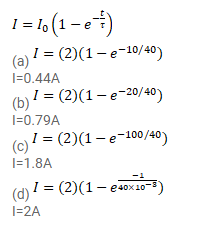# The time constant of an LR circuit is 40ms.

Question:

The time constant of an LR circuit is $40 \mathrm{~ms}$. The circuit is connected at $t=0$ and the steady-current is found to be $2.0 \mathrm{~A}$. Find the current at

(a) $t=10 \mathrm{~ms}$,

(b) $t=20 \mathrm{~ms}$,

(c) $t=100 \mathrm{~ms}$ and

(d) $t=1 \mathrm{~s}$.

Solution: